# Developments and Applications of Biostatistical Time Series: A Review

Shelton Peiris*1 and Tim Swartz2

1Visiting from School of Mathematics and Statistics, The University of Sydney, Australia

2Department of Statistics and Actuarial Science, Simon Fraser University, Canada

Received Date: November 27, 2019  Published Date: December 16, 2019

#### Abstract

Many data sets from medical science are available as time series, especially the records in births, epidemiology, fatal accidents, medical expenses etc, where the information are collected over time. This paper gives a short review of time series methods which have been used in medical research.

Keywords: Time series; Autoregression; Serial correlation; Stationarity; ARMA; Forecasting

#### Introduction

A time series is a collection of measurements recorded through a suitable time scale. Although this time scale may not be equally spaced, many applications are based on equally spaced time series data. It is known that time series data are generated when a population or an important phenomenon is monitored over time. Many time series analysts use the family of autoregressive integrated moving average (ARIMA) as it enjoys fruitful applications in modelling and forecasting of time series data (ie. serially or autocorrelated data) arise in almost all social sciences. Each member of this family assumes that future values of the series have a clearly defined dynamic parametric relationship which involves both current and past values together with a random noise. A number of extensions to this ARIMA family have been developed to model time series data not following the standard assumptions. A reason for this is that accurate modelling and analysis are useful in practice to estimate potential future observations or forecast values.

Many countries around the world use time series methodology to increase the quality of human life in health, epidemiology, national planning, controlling mortality etc as they a ect their developments. Planning and forecasting of population or migration are also essential for allocation of funds for social services of nations. Therefore, developing good time series models to best suits data sets and use them for accurate forecasting is essential in applications. This review paper is dedicated and focused on a short review of modeling and forecasting through popular time series models highlighting some potential applications through the R statistical software package.

#### Popular Time Series Models in Practice

Suppose that we have observed a time series of n observations on { Xt } following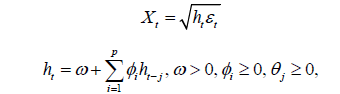where L is the lag operator such thatis a sequence of uncorrelated random variables,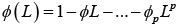is a polynomial of order p andpolynomial of order q: Since Xt has integrated at order d; (2.1) is known as an autoregressive integrated moving average of order ( p, d,q) and is abbreviated by ARIMA( p, d,q) : Although it isexpressed in a very general form, in many applications, we need integers of ( p, d,q) such that 0 ≤ p, d,q ≤ 2 , and making ieasy to apply in practice. Stationarity of the series and the orders p; d; q can be identified through the time series plot, autocorrelation (acf) and the partial autocorrelation (pacf) of the data. The time series plot tells whether it needs prior transformations and/or differencing to make it to a stationary series { Y t }Some possible models are:

• If the acf of { Yt } (transformed series) or{ Xt } (original series) decays very quickly (exponentially), then the time series is called ‘short memory’ and use the standard ARMA modelling techniques to and the locallybest possible model.

• If the acf of { Yt } and{ Xt } decays very slowly (hyperbolically), then the time series is called ‘long memory’ and use the theory of fractional differencing together ARMA techniques to and the best possible model. The corresponding model is similar to that of (2.1) with a fractional degree of differencing 0 < d < 0:5: This family is known as autoregressive fractionally integrated moving average or ARFIMA(p,d,q).

Replacing the operator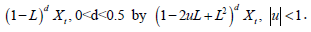there is a very general long memory time series known as Gegenbauer ARMA or GARMA(p,d,q; u) with many applications infinance and biostatistics. See for example, Dissanayake et al. (2018) and references therein.

• For certain time series data sets, the acf is not significant for the original data on t X but the acf of 2 t X is very significant. Such data can be modelled using the theory on generalized autoregressive conditional heteroscedastic (GARCH) models. This family of GARCH (p; q) is given byWherecomprises of a stationary sequence of independent and identically

distributed random variables satisfying zero mean, unit variance and ht is the conditional variance of { Xt } given the history;##### Illustrations

Below we illustrate these three cases in applications. Notice that acf of the last plot shows no serial correlation of data, however, Data2 (or Data2) contains significant acf values (Figure 1).

Now we look at an application of ARIMA modelling using a biostatistical time series data set.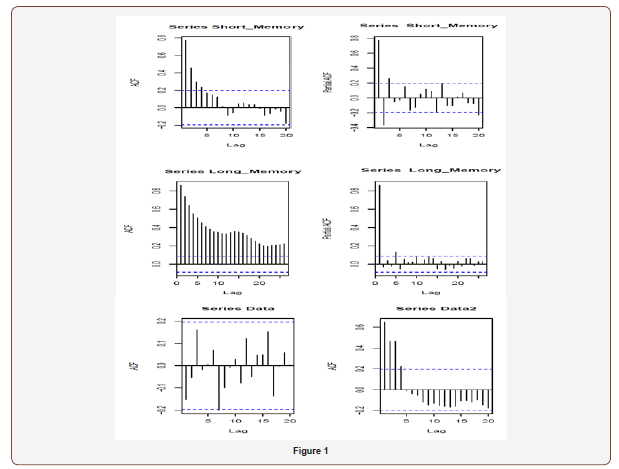#### ARIMA Modelling in Biostatistics

This section considers an application from annual incidences of stomach cancer in Australia from 1982 to 2015 (available in Cancer data in Australia https://www.aihw.gov.au/) to illustrate the procedure. The time series plot of data shows a slight upward trend. We conduct the analysis based on both the original and lag 1 differenced data and select the best possible model from a pool of all potential models. Time series plots of cancer cases (original data) and Differenced data (lag one differenced data) together with corresponding acf and partial pacf are given below: (Figure 2).Based on the above plots, suggest the following ARMA models: Table 1

Table 1: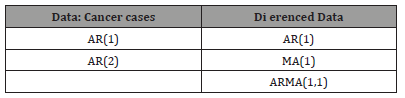Estimating each model using R give the following summary (values in parentheses are the corresponding estimated standard errors) Table 2:

Table 2: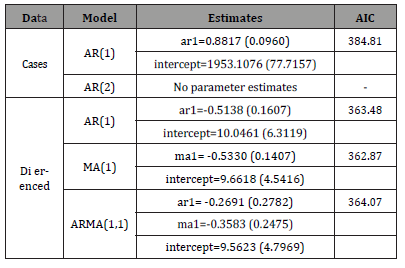This tells us that the differenced MA(1) model for the differenced data is the best among those have been suggested since it has the smallest aic value. Let { Xt } be the original series and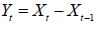represents the differenced series. Therefore, the best fitted model for { Yt } iswhere {et} is the associated noise.

Further applications of ARIMA modelling in biostatistical time series can be found in Cox and Solomon (1988), Zeger et al. (2004), Zeger et al. (2004), Perera et al. (2008), Zollar et al. (2016), Siskina and Siaulys (2016), Yousefzadeh-Chabok et al. (2016), Ferenci (2017), Fried et al. (2017), Xie (2017), Pavareh et al. (2018),Dissanayake et al. (2018), Earnest (2019) and references theirin.

None.

#### Conflict of Interest

No conflict of interest.

Article Details
Citation
Keywords
Scroll to
Scroll to Top